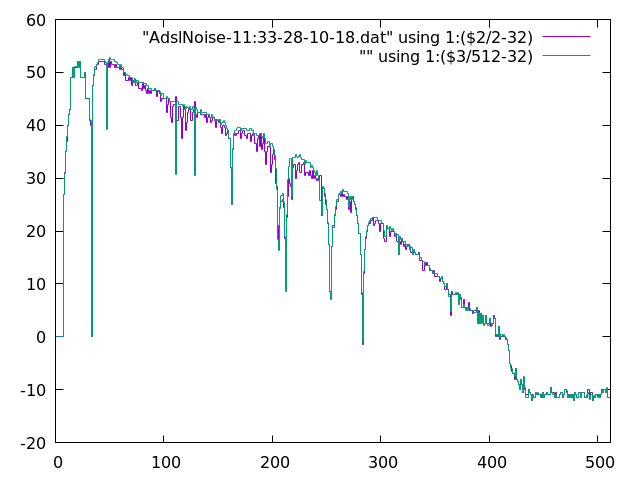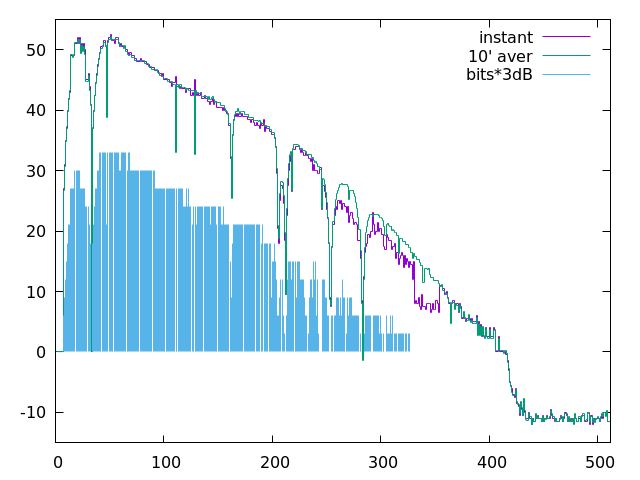One of the reasons for switching to open-wrt in my adsl router is that I have intermittent noise on my line. So I wanted a script that monitored the line, and emailed the statistics whenever noise appears. The current draft of this script is below in case its helpful the anyone.

It samples the up and down stream SNR on the ADSL, split down into its 512 frequencies. The email contains an instantaneous measure of this as well as a rolling average over 256 points (so with 10s sampling, thats about 40 minutes).

I detect the noise as the difference between the two, this is technically a lowest order z^{-1} high pass filter with frequency cut off of about 1 / 40 minutes. This is called delta in the code. I have two tests currently on delta, firstly the two bins which shows the greatest gain or loss in signal. Also the total sum of all the deltas. The first picks up noise in a single channel, that mainly seems to detect radio stations changing their output. The sum is a more distributed test across all channels.

The rolling average is a mess btw, ash doesn't seem to do arrays that I could work out, so the rolling average is stored in a 2048 character string consisting of 4 digit hex.

I hope it gives others ideas, the noise detection needs work I know -so ideas welcome ...

``````#!/bin/ash
rav=''

while true; do

# lock the pipe
lock /var/lock/dsl_pipe
# Downstream SNR
echo g997sansg 1 > /tmp/pipe/dsl_cpe0_cmd
linedsnr=`sed -n '2 p' < /tmp/pipe/dsl_cpe0_ack`
# Upstream SNR
echo g997sansg 0 > /tmp/pipe/dsl_cpe0_cmd
lineusnr=`sed -n '2 p' < /tmp/pipe/dsl_cpe0_ack`
# unlock the pipe
lock -u /var/lock/dsl_pipe
# Merge the two - 32bits upstream, 512 total bits
linesnr=\${lineusnr:1:95}\${linedsnr:96:1440}
ravnxt=''
ravlst=\${rav}
deltamax=0
deltamin=0
deltasum=0
for bit in \${linesnr}
do
ravtxt=\${rav:0:4}
rav=\${rav:4:2048}
if [ -z \${ravtxt} ]; then
delta=0
ravnxt=\${ravnxt}\$( printf '%4x' \$(( 0x\${bit} << 8 )) )
#	ravnxt=\${ravnxt}\${bit}'00'
#	echo -n "\${bit} "
else
delta=\$(( 0x\${bit} -((0x\${ravtxt} + 128) >> 8) ))
ravnxt=\${ravnxt}\$( printf '%4x' \$(( 0x\${ravtxt}+\${delta} )) )
fi
if [ \${delta} -gt \${deltamax} ]; then
deltamax=\${delta}
fi
if [ \${delta} -lt \${deltamin} ]; then
deltamin=\${delta}
fi
deltasum=\$(( \${deltasum} + \${delta} ))
done
echo -n 'delta var : [ '\${deltamin}' : '\${deltamax} '] Sum : '\${deltasum}' '
date
rav=\${ravnxt}
# if [ \${deltamin} -lt -30 -o \${deltamax} -gt 30 ] ; then // this gets stations //
if [ \${deltasum} -lt -300 -o \${deltasum} -gt 300 ] ; then
# Noise over limit so send an email
echo -n '# ' > /tmp/email.txt
date >> /tmp/email.txt
i=1
for bit in \${linesnr}
do
ravtxt=\${ravlst:0:4}
ravlst=\${ravlst:4:2048}
echo \${i} \$( printf '%d' "0x\${bit}" ) \$( printf '%d' "0x\${ravtxt}" ) >> /tmp/email.txt
i=\$(( \${i} + 1 ))
done
fi
sleep 10

done
``````

Nice!

You might want to use:

`. /lib/functions/lantiq_dsl.sh ; dsl_cmd g997sansg 1`
`echo g997sansg 1 > /tmp/pipe/dsl_cpe0_cmd`

I believe that way there is better locking around the command (but I have not looked too closely and might be way off).

My code was a copy of `/sbin/dsl_cpe_pipe.sh` although I copied it from the original firmware, that has the same code.

Looking at your file, I guess doing it that way would make it more general - as it seems to do many chipsets.

Well one night up, and its clear that the lower SNR at night time causes an issue. As the SNR drops, the rolling filter will lag, and that gives stress on the delta - and is enough to trigger an email, e.g. one like:And problem is with a step function change like this - it takes 40 odd minutes to escape from the fliter - so get emails every 10secs for quite a time period.

The min/max does similar things when radio stations change their output.

I'll need to work out a fliter that triggers on a step change just one. Alas that isn't obvious, as it implies a non causal filter. ANyway gives some code to work on ...

Find below v2 of the code, with work on the algorithms. I tried using the least causal high pass fliter, which is the difference between subsequent samples - that increases response to white noise by a factor of 40% or so. In practice it didn't help much, and was noise as anything. So went back to the rolling average method. A 256 point rolling average is about 40 minutes at samples every 10s, and that was giving problems with the lower margins overnight - the 40 minutes gave too much memory of the daylight conditions ...
So backed off the rolling average, 64 point gives about 10 minutes, which is far less senstive. This meant I could avoid the alarm triggering to quickly. Now it flags noise about once a day, and the graphs produced look like real noise. E.g. see bellow:One problem with the code is

``````\$((`date +%S`%10))
``````

This gives an -ash arithemtic syntax error something like 1 in 100 times. As a result the code to take samples on multiples of 10s, doesn't work. Instead it sleeps for 4s each loop, which gives about 10s samples.

``````#!/bin/ash
rav=''
lst=''
fitno=6 # this gives the rolling average length in range 1-8
# with 10s samples
# 1 =    20"
# 2 =    40"
# 3 =  1'20"
# 4 =  2'40"
# 5 =  5'20"
# 6 = 10'40"
# 7 = 21'20"
# 8 = 42'40"
offst=\$(( 1 << (\${fitno} - 1) )) # this is used for rounding in >>\${fitno}
zero=\$((64<<\${fitno})) # this rerepesents zero in the rolling average

while true
do
sleep 4
# problem with this code is that \$((`date +%S`%10))
# gives an "arithmetic syntax error" at times
# cryptic code to want till next 10s multiple
#st=\$((10-(`date +%S`%10)))
#if [ \${st} -gt 0 ] ; then
#    sleep \${st}
#fi
# lock the pipe
lock /var/lock/dsl_pipe
# Upstream SNR
echo g997sansg 0 > /tmp/pipe/dsl_cpe0_cmd
lineusnr=`sed -n '2 p' < /tmp/pipe/dsl_cpe0_ack`
# Downstream SNR
echo g997sansg 1 > /tmp/pipe/dsl_cpe0_cmd
linedsnr=`sed -n '2 p' < /tmp/pipe/dsl_cpe0_ack`
# Upstream bits
echo g997bansg 0 > /tmp/pipe/dsl_cpe0_cmd
lineubit=`sed -n '3 p' < /tmp/pipe/dsl_cpe0_ack`
# Downstream SNR
echo g997bansg 1 > /tmp/pipe/dsl_cpe0_cmd
linedbit=`sed -n '3 p' < /tmp/pipe/dsl_cpe0_ack`
# unlock the pipe
lock -u /var/lock/dsl_pipe
# Merge the two - 32bits upstream, 512 total bits
linesnr=\${lineusnr:1:95}\${linedsnr:96:1440}
linebit=\${lineubit:1:95}\${linedbit:96:1440}
ravnxt=''
deltamax=0
deltamin=0
deltasum=0
posi=0
ravi=0
snrusum=0
snrdsum=0
# create the file for the email
echo -n '# ' > /tmp/email.txt
date >> /tmp/email.txt
i=1
while [ \${i} -le 512 ]
do
# For the various strings, which contain arrays, look up data by ofsets
snr=\${linesnr:\${posi}:2}
snrd=\$(printf '%d' "0x\${snr}")
bit=\${linebit:\${posi}:2}
bitd=\$(printf '%d' "0x\${bit}")
posi=\$((\${posi}+3))
ravtxt=\${rav:\${ravi}:4}
ravi=\$((\${ravi}+4))

if [ -z \${ravtxt} ]; then
ravd=0
delta=0
ravnxt=\${ravnxt}\$( printf '%04x' \$((\${snrd}<<\${fitno})) )
else
ravd=\$(printf '%d' "0x\${ravtxt}")
delta=\$(( \${snrd} - ((\${ravd}+\${offst})>>\${fitno}) ))
ravnxt=\${ravnxt}\$( printf '%04x' \$((\${ravd}+\${delta})) )
fi
if [ \${delta} -gt \${deltamax} ]; then
deltamax=\${delta}
fi
if [ \${delta} -lt \${deltamin} ]; then
deltamin=\${delta}
fi
deltasum=\$(( \${deltasum} + \${delta} ))
if [ "\${snr}" != "FF" -a \${snrd} -gt 64 ] ; then
if [ \${i} -le 32 ]; then
snrusum=\$((\${snrusum}+(\${snrd}-64)/6))
else
snrdsum=\$((\${snrdsum}+(\${snrd}-64)/6))
fi
fi

if [ "\${snr}" = "FF" -o "\${ravtxt}" = "" ] ; then
echo \${i} "64 \${zero} 0" >> /tmp/email.txt
else
echo \${i} \${snrd} \${ravd} \${bitd} >> /tmp/email.txt
fi
i=\$((\${i}+1))
done
echo -n 'ra: [ '\${deltamin}' : '\${deltamax} '] S: '\${deltasum}' : '\$((\${snrusum}*8625/2048))' '\$((\${snrdsum}*8625/2048))' '
date
rav=\${ravnxt}
if [ \${deltasum} -lt -600 -o \${deltasum} -gt 600 ] ; then
mailsend -f router@you.at.your.email -t you@you.email.address -smtp isp.relay.your.isp -port 587 -sub "ADSL Noise File : \${deltasum} : `date`" -msg-body /tmp/email.txt
fi
done

``````